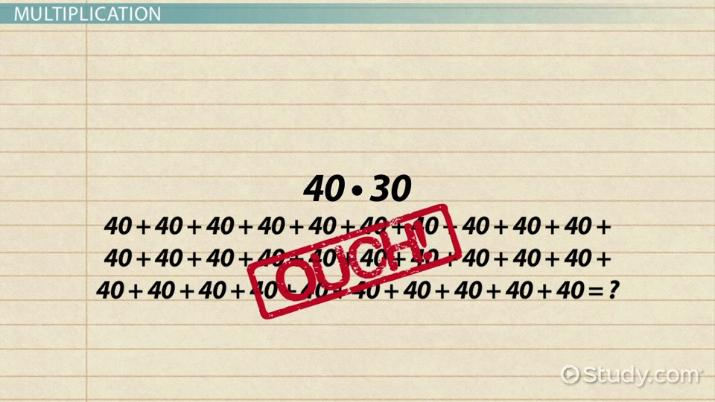# How to Multiply Numbers Ending in Zeroes

Lesson Transcript
Instructor: Yuanxin (Amy) Yang Alcocer

Amy has a master's degree in secondary education and has taught math at a public charter high school.

In this lesson, you will learn how to multiply numbers that end in zeroes. You will see that multiplying numbers that end with zeroes is an easy process that requires some counting.

## Multiplication

In this lesson, we will talk about multiplication; multiplication with numbers that end in zeroes. Multiplication is repeated addition. It is telling you that you have several groups of a certain number to add together. So, 4 * 3 (four times three) means that you have three groups of 4 that you need to add together: 4 + 4 + 4.

So what happens if we add zeroes behind our numbers? Like, what if we multiply 40 * 30 together? You can see that this means we have thirty groups of 40. This is quite a bit to add together manually. So what can you do? You can follow this little trick for multiplying with numbers that end with zeroes.An error occurred trying to load this video.

Try refreshing the page, or contact customer support.

Coming up next: How to Complete the Multiplication Sentence

### You're on a roll. Keep up the good work!

Replay
Your next lesson will play in 10 seconds
• 0:01 Multiplication
• 0:37 Multiplying by Zeroes
• 1:24 Examples
• 1:42 In a Word Problem
• 2:28 Lesson Summary
Save Save

Want to watch this again later?

Timeline
Autoplay
Autoplay
Speed Speed

## Multiplying by Zeroes

The trick involves counting the zeroes. So, to multiply 40 * 30, you would multiply the 4 * 3 and then count the zeroes. Your answer is what you get from multiplying the non-zero numbers and then writing the number of zeroes you have counted.

So, for the problem 40 * 30, your non-zero numbers are 4 and 3. You have two zeroes, one from the 40 and one from the 30. Then your answer comes from multiplying 4 *3 and adding the number of zeroes behind it. So, 4 * 3 is equal to 12, so 40 * 30 is equal to 12 with two zeroes after it: 1,200.

This trick will serve you well when you are taking math tests and you want an easy and quick way to answer these problems. Let's take a look at a couple more example problems.

## Example

Multiply 100 * 20.

In this problem, we see that our non-zero numbers are 1 and 2. How many zeroes do we have? We have three: two from the 100 and one from the 20. So, your answer is 1 * 2 = 2 with three zeroes behind it: 2,000.

## In A Word Problem

Now, what if we had a word problem?

To unlock this lesson you must be a Study.com Member.

### Register to view this lesson

Are you a student or a teacher?